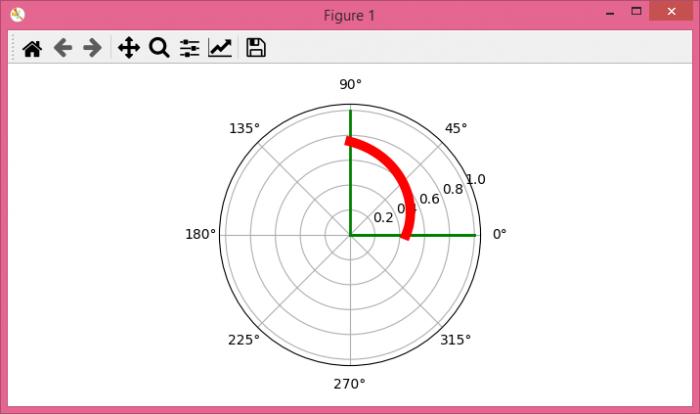# How to curve text in a polar plot in matplotlib?

MatplotlibPythonData Visualization

To curve text in a polar plot in matplotlib we can take the following steps

## Steps

• Set the figure size and adjust the padding between and around the subplots.

• Create a new figure or activate an existing figure.

• Add an 'ax' to the figure as part of a subplot arrangement.

• Plot the line with some degree, color='green' and linewidth=2.

• Create x and y data points, with some curve and plot them using plot() method.

• To display the figure, use Show() method.

## Example

from matplotlib import pyplot as plt
from scipy.interpolate import interp1d
import numpy as np

plt.rcParams["figure.figsize"] = [7.00, 3.50]
plt.rcParams["figure.autolayout"] = True

fig = plt.figure()

for degree in [0, 90, 360]:

for curve in [[[0, 90], [0.45, 0.75]]]:
plt.show()